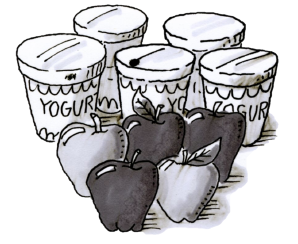### Home > CC2 > Chapter 5 > Lesson 5.2.6 > Problem5-88

5-88.Lucas is having yogurt and an apple for a snack. There are five containers of yogurt in the refrigerator: three are raspberry, one is vanilla, and one is peach. There are also two green apples and three red apples.

1. If he reaches into the refrigerator to get a yogurt without looking, what is the probability that Lucas will choose a raspberry yogurt?

How many raspberry yogurts are there out of the total number of yogurts in the refrigerator?

2. What is the probability that he will choose a red apple if it is the first item he selects?

How many red apples are there out of all the apples?

3. What is the probability that Lucas will eat a raspberry yogurt and a red apple?

Lucas' choices for the apple and yogurt are independent because the outcome for choosing one type of food will not affect his choice when choosing for the other type.

To find the probability of two independent events both occurring, find each probability separately and then multiply their probabilities.

$\frac{3}{5}\cdot\frac{3}{5}=\frac{9}{25}$